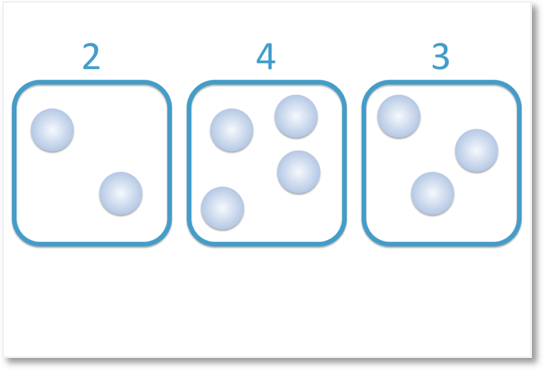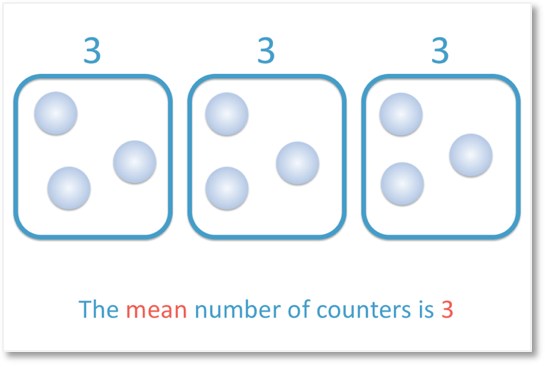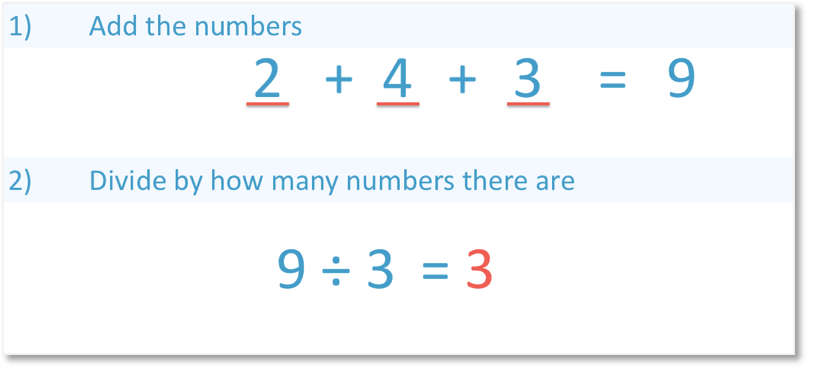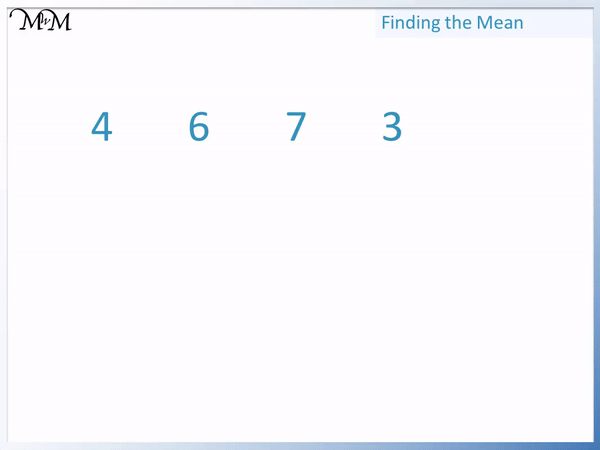# How to Find the Mean

How to Find the Mean• The mean is an average.
• We will find the average number of counters in these 3 groups.
• To find the mean, we add up the numbers to find the total.
• 2 + 4 + 3 = 9.
• We now share the counters out equally between the 3 groups.
• 9 ÷ 3 = 3
• The mean number of counters is 3.

To find the mean we add the numbers up and divide this total by how many numbers there are.• To find the mean of a set of numbers, add the numbers and divide by how many numbers there are.
• Adding the numbers we have: 2 + 4 + 3 = 9.
• There are 3 numbers in total, which are ‘2’, ‘4’ and ‘3’.
• 9 ÷ 3 = 3.
• The mean of the numbers is 3.Supporting Lessons# How to Find the Mean

The mean is an average of a set of numbers.

To calculate the mean of a set of numbers, use the following steps:

1. Add the numbers to make a total.
2. Divide this total by how many numbers there are.

First we will look at what the mean is.

Below, we have three groups of counters.We will share these counters equally between the three groups.

We begin by grouping all of the counters together as a total.We then divide them equally across the three groups.There are now 3 counters in each group.

The mean is 3.When teaching the mean, we can introduce the idea of an average through physical objects like counters. However once the idea is understood, it is best to teach the mean with mathematical steps.

We will look at the same example but this time we will use the steps for finding a mean.2 + 4 + 3 = 9

Step 2: Divide by how many numbers there are.

There are three numbers (‘2’, ‘4’ and ‘3’), so we divide 9 by 3.

9 ÷ 3 = 3

Therefore, the mean is 3.Here is an example of finding the mean of the four numbers: 4, 6, 7 and 3.

The first step to find the mean is to add the numbers.

It helps to look for number bonds. For example, we have 4 + 6 and 7 + 3. Both of these pairs of numbers add to make 10.

4 + 6 + 7 + 3 = 20

The second step is to divide this resulting total by how many numbers there are.

There are four numbers: ‘4’, ‘6’, ‘7’ and ‘3’.

We divide 20 by 4.

20 ÷ 4 = 5The mean of this set of numbers is 5.

In this next example we are calculating the mean of five numbers: 6, 0, 3, 4 and 2.

The first step is to add the numbers.

We can add 6 + 4 to make 10 and then add on the 3 and the 2.

6 + 0 + 3 + 4 + 2 = 15.

The next step is to divide by how many numbers there are. There are five numbers.

A common question is whether we include the zero as one of the numbers. We do include the ‘0’. If we do not include the zero, it will give us a different answer.

15 ÷ 5 = 3And so, the mean of this set of data is 3.Now try our lesson on Finding the Median where we learn how to calculate the median.error: Content is protected !!